# How to Use the data Validation Input Message as a Cell Comment in Excel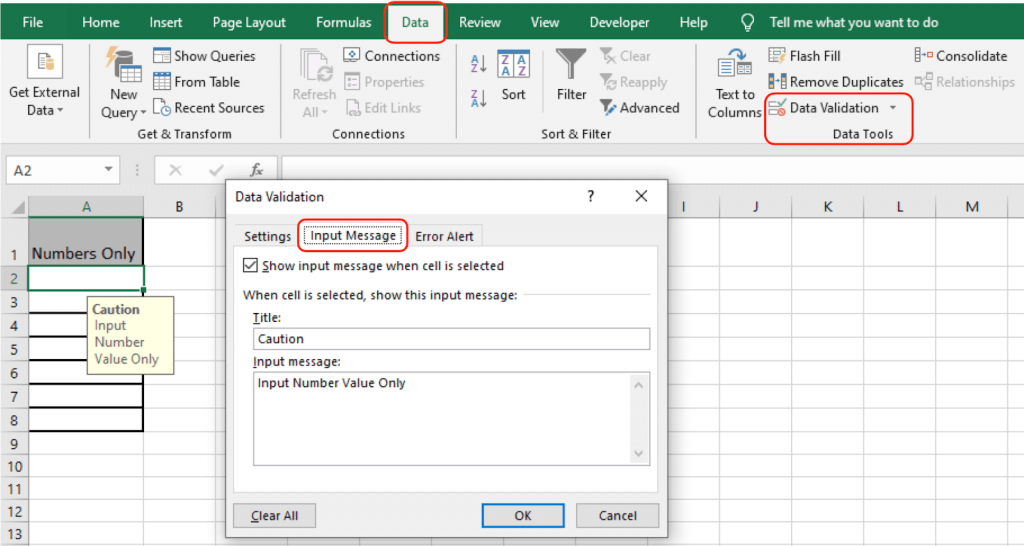In this article, we will learn How to Use the data Validation Input Message as a Cell Comment in Excel.

Scenario:

Data Validation is the tool that is used to restrict the entry in a range or data so that we can define which type of data should be entered in the sheet. It guides the user to input the type of option which is necessary for your worksheet. Here the problem is, we need to input message as caution. We add a caution message to let the user know what values to enter. Let's learn more about Data validation input message in Excel here.

Data Validation Input Message in Excel

Go to Data > Data validation arrow > Data validation > Input title > Input message > Click Ok.

Note: You can also customize the warning message from the Error alert message.

Example :

All of these might be confusing to understand. Let's understand how to use the function using an example. Here we will apply a data validation on the cell to allow the user to input any value which is a number. Below is the snapshot how to use data validation.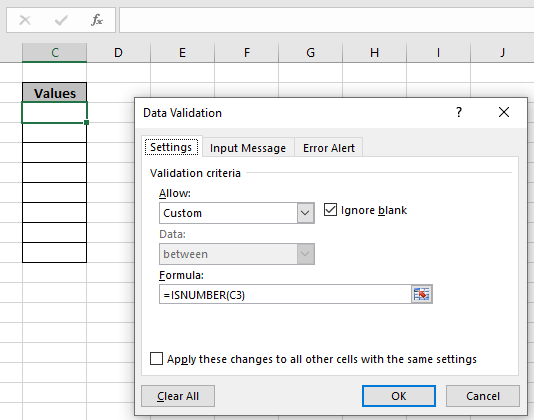Here you noticed after Select Custom from Allow method list which allows you to use the formula in cell. Click Ok to apply.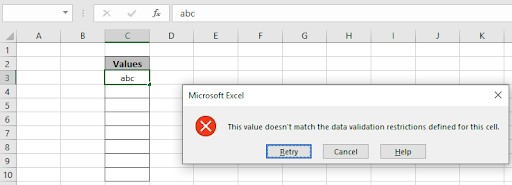We tried typing the text value as we enter a text value, it throws an error "This value doesn't match the data validation restrictions defined for this cell".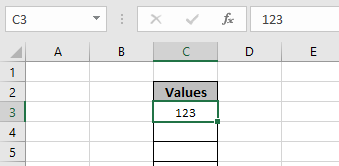Now, we tried the number value in the cell and the cell accepts the number value as shown in the above image. You can also customize the error message and alert message to let users know what values are prohibited. Edit alert and message as shown below.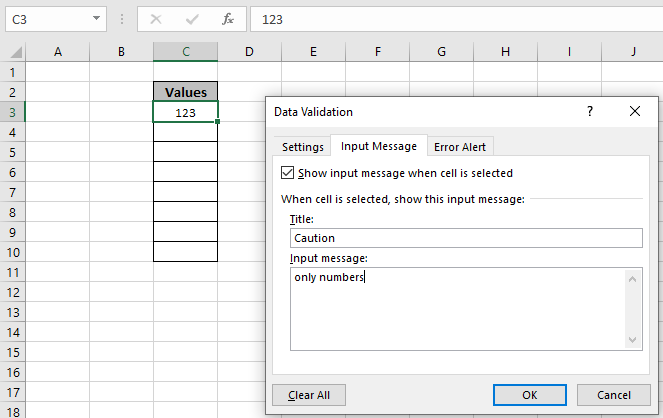Input Alert message be typed in Input Message tab as shown in above image.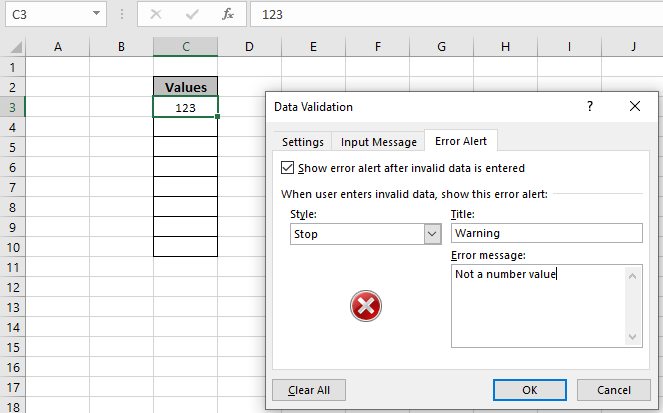An Error Alert message be used in the Error Alert tab. Now click OK to get these changes.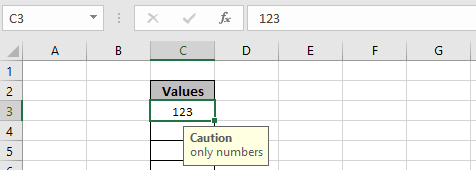As you can see the Caution alert in the yellow box hanging below C3 cell. Now you can copy the Data validation format to the rest of the required cell using Ctrl + D.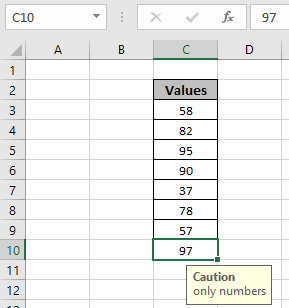As you can see, now these cells can be used to allow numbers only. Now learn how to perform Data validation using VBA

Alternate way using VBA

We have countries’ names in the D column, and in cell A1, we want to create a drop down filter for countries’ names.

We want to highlight the cell A1 and make the drop down list to countries’ names and put the message to let the users know which entries need to be entered in the range.

Follow below given steps and code:-

Open the VBE page to press the key Alt+F11.

Insert the module to write the code.

Write the below mentioned code:-

 Sub DropDownFilter() Dim lastrow As Long lastrow = Worksheets("sheet1").Cells(Rows.Count, "D").End(xlUp).Row     With Range("A1:A10").Validation     .Add Type:=xlValidateList, AlertStyle:=xlValidAlertStop, Operator:=xlBetween, Formula1:="=\$D\$2:\$D" & lastrow     .IgnoreBlank = True     .InCellDropdown = True     .InputTitle = "Message"     .InputMessage = "Enter only countries name"     Range("A1:A10").Interior.ColorIndex = 37     End With End Sub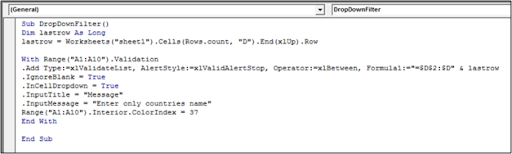Add the code as shown above and press f5 from the keyboard to enable the change on the sheet.

First we had defined all the variables, then we had created the range which would help to create the dynamic drop down filter. Similarly, we had defined the input title and Input message. And then we had defined the destination where we wanted to create the drop down list and then we would fill the cell with color.

You can use a formula to allow text value only i.e. =ISTEXT(cel_ref). You can use any formula that returns True or False in the formula bar of the data validation tool.

Here are all the observational notes regarding using the formula.

Notes:

1. Apply the data validation tool to restrict the user to input invalid value, which doesn't satisfy the condition.
2. Data validation tool can work with numbers and text both.

Hope this article about How to Use the data Validation Input Message as a Cell Comment in Excel is explanatory. Find more articles on calculating values and related Excel formulas here. If you liked our blogs, share it with your friends on Facebook. And also you can follow us on Twitter and Facebook. We would love to hear from you, do let us know how we can improve, complement or innovate our work and make it better for you. Write to us at info@exceltip.com.

Related Articles :

Data Validation in Excel : Data Validation is a tool used to restrict users to input value manually in cell or worksheet in Excel. It has a list of options to choose from.

Way to use Vlookup function in Data Validation : Restrict users to allow values from the lookup table using Data validation formula box in Excel. Formula box in data validation allows you to choose the type of restriction required.

Restrict Dates using Data Validation : Restrict user to allow dates from a given range in cell which lays within Excel date format in Excel.

How to give the warning messages in Data Validation : Restrict users to customize input information in the worksheet and guide the input information through error messages under data validation in Excel.

Create Drop Down Lists in Excel using Data Validation : Restrict users to allow values from the drop down list using Data validation List option in Excel. List box in data validation allows you to choose the type of restriction required.

Popular Articles :